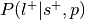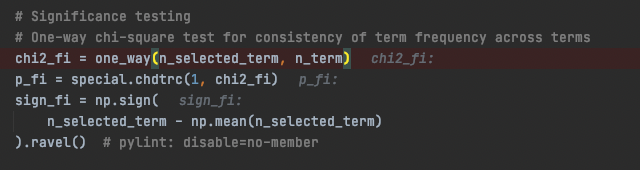# Nimare Decoding Results

Hi,

We are aiming to decode some .nii images with Nimare/Neurosynth. I followed the [„decoding“] example on the Nimare Webpage and was able to successfully implement the NeurosynthDecoder. Now, I have some questions regarding the interpretation of the results. On the Neurosynth Webpage it is stated that the terms „uniformity" and „association test“ are replacing the forward and reverse infererence analysis. The Nimare results table however is displaying probReverse and probForward values (e.g.). Is there anywhere a more elaborate documentation on how you calculate these values via Nimare? Or are they basically just calculated with bayesian statistics (meaning probReverse is the posterior probability)?

Ole

Hi Ole,

You’re right- the documentation for the decoding methods is lacking, mostly because they haven’t gotten a lot of use yet. I can try to make improving the documentation on those methods a priority.

The “reverse inference” results from the decoder correspond to the association test, while the “forward inference” results correspond to the uniformity test. In fact, the NeurosynthDecoder is just a reformulation of the MKDA Chi2 approach Neurosynth uses for its meta-analyses.

Yes, the probability values are posterior probabilities. The probForward values represent the probability of study selection given the presence of the label and the prior probability of having the label, while the probReverse values represent the probability of presence of the label given selection and the prior probability of having the label.

The prior probability of having the label in the Neurosynth approach is just a single a priori probability, by default 0.5 (50% chance of a brain experiencing the brain state described by the label). “Selection” refers to whatever procedure you use to identify the subset of studies you’re using to decode; perhaps the most common example being studies which report at least one coordinate in an ROI.

Best,
Taylor

Hi Taylor,

Many thanks for the quick response!!

You’re right- the documentation for the decoding methods is lacking, mostly because they haven’t gotten a lot of use yet. I can try to make improving the documentation on those methods a priority.

Sounds really good, thank you!

Yes, the probability values are posterior probabilities. The probForward values represent the probability of study selection given the presence of the label and the prior probability of having the label, while the probReverse values represent the probability of presence of the label given selection and the prior probability of having the label.
The prior probability of having the label in the Neurosynth approach is just a single a priori probability, by default 0.5 (50% chance of a brain experiencing the brain state described by the label). “Selection” refers to whatever procedure you use to identify the subset of studies you’re using to decode; perhaps the most common example being studies which report at least one coordinate in an ROI.

Just to make sure, I got it right. This basically means the probReverse is:
„ ProbForward = P(Study Selection* | Label) ; prior = p(Label)“
*based on „at least one activation within an Roi“ within Roi

And the reverse inference is:
„ProbReverse = P(Label | Study Selection*) ; prior = p(Label)“
*based on „at least one activation within an Roi“ within Roi

Since we try to make more sense of the decoded data, we aim to calculate the Bayes Factor of the ProbReverse Values based on the posterior probability and the prior (as described in Poldrack, 2006, and in more detail in Goodman, 1999), it would be highly appreciated to get to know, whether the Nimare/Neurosynth-approach uses actually the Bayesian statistics to this effect.

I am just wondering, because the aforementioned uniformity and association tests seem to produce z-values. I am still not entirely sure how you actually calculate the probReverse and probForward values (since it is stated on the Neurosynth FAQ that the uniformity and association analysis differ from the Bayesian estimation analysis).

Via BrainMap the probReverse (e.g) is calculated according to Bayes Rule with P(Term|Activation = P(Activation|Term)*P(Term)/P(Activation). Where P(Activation|Term) = Experiments per specific Term within Roi/Experiments per specific Term in whole database. P(Activation) = Number of Experiments in Roi/Number of all Experiments in database. And p(Term) = Number of a specific Term in database/Number of all Experiments in database.

Would it be possible to get a documentation where it is shown, how probReverse via Neurosynth is calculated in more detail (e.g. as I did for BrainMap)? Just to make sure that we interpret the results of the decoding correctly.

Best,

Ole

I have detailed descriptions of the two approaches, with a little comparison, in appendices for a manuscript I’m working on. I can take the text and formulae from my manuscript and put them in NiMARE’s documentation.

Hi Talyor,

that would be great, thank you!

Best,

Ole

Dear Taylor,
I am facing the same doubts as Ole. Please, would you tell me where I can find the NiMARE document describing the two different approaches? I am sorry but I cannot find it.
Thank you so much for your help,
Stefania

I’ve added a page about decoding approaches to the NiMARE documentation: `Meta-analytic functional decoding`. It’s currently bare bones, with only the step-by-step descriptions of the BrainMap and Neurosynth discrete decoding methods, but hopefully it will help you. I will try to expand the documentation over the next few weeks.

Thank you very much Taylor!

Hi Taylor,

I’m working on the functional decoding with my own data, and I’m confused about the statistical significance of the results. The decoding using NiMARE returned ‘probReverse’ and ‘pReverse’, but I do not figure out which ‘proReverse’ survive the fdr correction, i.e are these ‘pReverses’ the p-values which already be corrected?

Ray

`probReverse` refers to the posterior probabilities for the reverse inference analysis, rather than p-values. In our decoding documentation, `probReverse` is. I hope that helps!

Hi Taylor,

My apology for the ambiguous statement. I learnt the meaning of the ‘probReverse’ from the docs of NiMARE. However, I am not sure whether the returned ‘probReverse’ was corrected for the multiple comparisons concern. Specifically, I applied the decoder to the region of interest with FDR-correction method built in the code, and I got a Dataframe of decoding results with whole features in it. But, how can I extract those results which survived the multiple comparisons correction?

Best,
Ray

Ah, okay. Sorry about the confusion. probReverse isn’t corrected for multiple comparisons because the posterior probability is more a measure of effect size than statistical significance. If you used FDR correction in the decoder, then the pReverse, zReverse, pForward, and zForward values will be corrected for multiple comparisons, and you should apply a threshold based on one of those columns.

You could do something like the following:

``````significant_df = df.loc[df["pReverse"] < 0.05]
``````

Hi Taylor,

Thanks for the clarification. By such method to obtain significant results means that the p-values within Dataframe were already corrected and were FDR-p. Am I correct?

Best,
Ray

Hi Ray,

That’s right! The p- and z-values are corrected, but non-significant terms are not removed automatically.

Best,
Taylor

Hi Taylor,

In the code “discrete.py” the one-way chi-square produces p_fi.The two-way chi-square test produces p_ri.

Is p_fi refering to forward inference (thus, fi) and p_ri to reverse inference(thus, ri), which would mean, that in general the one way chi-square test solely produces forward inference p vals (and z-vals, though) and the two way chi-square test produces reverse inference p and z vals? Therefore, in the result DataFrame, the pReverse values for a given term are a product of the two-way chi square test (if applied, tested for multiple comparisons).

Just wanted to make sure i understood it correctly.

Best,

Ole

Hi Ole,

Yes, that’s correct!

Best,
Taylor

Thanks for the quick response!

Best,

Ole

Thanks for the clarification. My confusion has been resolved since then.Hi Taylor,

Could you please explain the difference between the z-value calculated from the NiMARE (“zReverse” decoding result) and the z-value calculated from NeuroSynth? NiMARE outputs signed z-value, does the negative z-value indicate negative correlation?

To give you some background about my question - We have replicated Daniel’s analysis using NiMARE. In the notebook, you can find that Daniel set a positive threshold for the z-value from NeuroSynth. However, NiMARE outputs have negative z-values. We’re wondering what negative z-value implies and if we should take the absolute value for the z-value before we apply a threshold.

Thanks!

Hi Xinhui,

The short answer is that NiMARE’s NeurosynthDecoder doesn’t use the same approach as Neurosynth’s Decoder when `method='roi'`. I get why the naming is a little confusing though. I actually have a NiMARE issue open about implementing the ROI approach (Add ROI association discrete decoding method from Neurosynth · Issue #437 · neurostuff/NiMARE · GitHub), but I haven’t gotten around to it yet.

From what I can tell, the ROI method extracts the average MA value within the ROI from each study in the database, and then correlates those values with the feature values (e.g., study-wise term tf-idf values), and then converts the resulting correlation coefficients to z-values. I assume that there are no negative z-values because Margulies et al. removed them with the following code (copied from the linked notebook):

``````thr = 3.1
vmin = 0
vmax = 15

tot = 5
data = decoder.decode([str('gradient_data/masks/volume_%02d_%02d.nii.gz' % (i * tot, (i * tot) + tot))
for i in xrange(0,100/tot)])
df = []
df = data.copy()
newnames = []
[newnames.append(('%s-%s' % (str(i * tot), str((i*tot) + tot)))) for i in xrange(0,len(df.columns))]
df.columns = newnames
df[df<thr] = 0  # <-- Here specifically
``````

I’m not sure why they did that, but I think that, without that step, there should be negative z-values as well.

NiMARE’s approach is a much more direct translation of Neurosynth’s meta-analysis method, applied to decoding. The reverse inference z-statistics come from a two-way chi-square test comparing (1) studies with each label and a peak within the ROI, (2) studies with each label and no peak in the ROI, (3) studies without the label and a peak in the ROI, and (4) studies without the label and no peak in the ROI. You can see the step-by-step description in NiMARE’s documentation here.

Negative z-values would indicate that there is a higher proportion of studies with the label among studies that do not have at least one peak in the ROI.

Best,
Taylor

1 Like